Courses

# Minimum Spanning Trees Computer Science Engineering (CSE) Notes | EduRev

## Computer Science Engineering (CSE) : Minimum Spanning Trees Computer Science Engineering (CSE) Notes | EduRev

The document Minimum Spanning Trees Computer Science Engineering (CSE) Notes | EduRev is a part of the Computer Science Engineering (CSE) Course GATE Computer Science Engineering(CSE) 2022 Mock Test Series.
All you need of Computer Science Engineering (CSE) at this link: Computer Science Engineering (CSE)

Kruskal’s Minimum Spanning Tree Algorithm

What is Minimum Spanning Tree?

Given a connected and undirected graph, a spanning tree of that graph is a subgraph that is a tree and connects all the vertices together. A single graph can have many different spanning trees. A minimum spanning tree (MST) or minimum weight spanning tree for a weighted, connected and undirected graph is a spanning tree with weight less than or equal to the weight of every other spanning tree. The weight of a spanning tree is the sum of weights given to each edge of the spanning tree.

How many edges does a minimum spanning tree has?

A minimum spanning tree has (V – 1) edges where V is the number of vertices in the given graph.

What are the applications of Minimum Spanning Tree?

Below are the steps for finding MST using Kruskal’s algorithm

1. Sort all the edges in non-decreasing order of their weight.

2. Pick the smallest edge. Check if it forms a cycle with the spanning tree
formed so far. If cycle is not formed, include this edge. Else, discard it.

3. Repeat step#2 until there are (V-1) edges in the spanning tree.

The step#2 uses  Union-Find alogrithm to detect cycle. So we recommend to read following post as a prerequisite.
The algorithm is a Greedy Algorithm. The Greedy Choice is to pick the smallest weight edge that does not cause a cycle in the MST constructed so far. Let us understand it with an example: Consider the below input graph.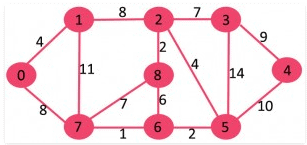The graph contains 9 vertices and 14 edges. So, the minimum spanning tree formed will be having (9 – 1) = 8 edges.

After sorting:
Weight   Src    Dest
1         7      6
2         8      2
2         6      5
4         0      1
4         2      5
6         8      6
7         2      3
7         7      8
8         0      7
8         1      2
9         3      4
10        5      4
11        1      7
14        3      5

Now pick all edges one by one from sorted list of edges
1. Pick edge 7-6: No cycle is formed, include it.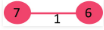2. Pick edge 8-2: No cycle is formed, include it.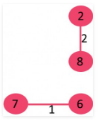3. Pick edge 6-5: No cycle is formed, include it.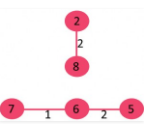4. Pick edge 0-1: No cycle is formed, include it.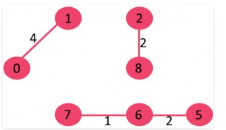5. Pick edge 2-5: No cycle is formed, include it.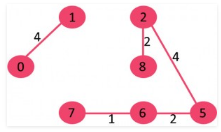6. Pick edge 8-6: Since including this edge results in cycle, discard it.

7. Pick edge 2-3: No cycle is formed, include it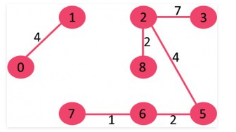8. Pick edge 7-8: Since including this edge results in cycle, discard it.

9. Pick edge 0-7: No cycle is formed, include it.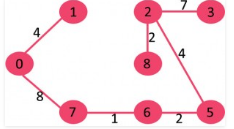10. Pick edge 1-2: Since including this edge results in cycle, discard it.

11. Pick edge 3-4: No cycle is formed, include it.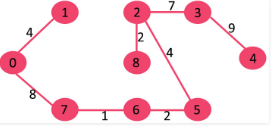Since the number of edges included equals (V – 1), the algorithm stops here.

// C++ program for Kruskal's algorithm to find Minimum Spanning Tree
// of a given connected, undirected and weighted graph
#include <stdio.h>
#include <stdlib.h>
#include <string.h>

// a structure to represent a weighted edge in graph
struct Edge
{
int src, dest, weight;
};

// a structure to represent a connected, undirected and weighted graph
struct Graph
{
// V-> Number of vertices, E-> Number of edges
int V, E;

// graph is represented as an array of edges. Since the graph is
// undirected, the edge from src to dest is also edge from dest
// to src. Both are counted as 1 edge here.
struct Edge* edge;
};

// Creates a graph with V vertices and E edges
struct Graph* createGraph(int V, int E)
{
struct Graph* graph = (struct Graph*) malloc( sizeof(struct Graph) );
graph->V = V;
graph->E = E;

graph->edge = (struct Edge*) malloc( graph->E * sizeof( struct Edge ) );

return graph;
}

// A structure to represent a subset for union-find
struct subset
{
int parent;
int rank;
};

// A utility function to find set of an element i
// (uses path compression technique)
int find(struct subset subsets[], int i)
{
// find root and make root as parent of i (path compression)
if (subsets[i].parent != i)
subsets[i].parent = find(subsets, subsets[i].parent);

return subsets[i].parent;
}

// A function that does union of two sets of x and y
// (uses union by rank)
void Union(struct subset subsets[], int x, int y)
{
int xroot = find(subsets, x);
int yroot = find(subsets, y);

// Attach smaller rank tree under root of high rank tree
// (Union by Rank)
if (subsets[xroot].rank < subsets[yroot].rank)
subsets[xroot].parent = yroot;
else if (subsets[xroot].rank > subsets[yroot].rank)
subsets[yroot].parent = xroot;

// If ranks are same, then make one as root and increment
// its rank by one
else
{
subsets[yroot].parent = xroot;
subsets[xroot].rank++;
}
}

// Compare two edges according to their weights.
// Used in qsort() for sorting an array of edges
int myComp(const void* a, const void* b)
{
struct Edge* a1 = (struct Edge*)a;
struct Edge* b1 = (struct Edge*)b;
return a1->weight > b1->weight;
}

// The main function to construct MST using Kruskal's algorithm
void KruskalMST(struct Graph* graph)
{
int V = graph->V;
struct Edge result[V];  // Tnis will store the resultant MST
int e = 0;  // An index variable, used for result[]
int i = 0;  // An index variable, used for sorted edges

// Step 1:  Sort all the edges in non-decreasing order of their weight
// If we are not allowed to change the given graph, we can create a copy of
// array of edges
qsort(graph->edge, graph->E, sizeof(graph->edge), myComp);

// Allocate memory for creating V ssubsets
struct subset *subsets =
(struct subset*) malloc( V * sizeof(struct subset) );

// Create V subsets with single elements
for (int v = 0; v < V; ++v)
{
subsets[v].parent = v;
subsets[v].rank = 0;
}

// Number of edges to be taken is equal to V-1
while (e < V - 1)
{
// Step 2: Pick the smallest edge. And increment the index
// for next iteration
struct Edge next_edge = graph->edge[i++];

int x = find(subsets, next_edge.src);
int y = find(subsets, next_edge.dest);

// If including this edge does't cause cycle, include it
// in result and increment the index of result for next edge
if (x != y)
{
result[e++] = next_edge;
Union(subsets, x, y);
}
}

// print the contents of result[] to display the built MST
printf("Following are the edges in the constructed MST ");
for (i = 0; i < e; ++i)
printf("%d -- %d == %d ", result[i].src, result[i].dest,
result[i].weight);
return;
}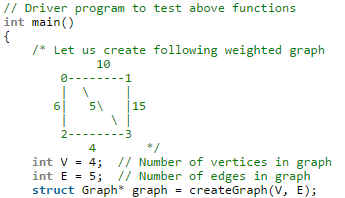graph->edge.src = 0;
graph->edge.dest = 1;
graph->edge.weight = 10;

graph->edge.src = 0;
graph->edge.dest = 2;
graph->edge.weight = 6;

graph->edge.src = 0;
graph->edge.dest = 3;
graph->edge.weight = 5;

graph->edge.src = 1;
graph->edge.dest = 3;
graph->edge.weight = 15;

graph->edge.src = 2;
graph->edge.dest = 3;
graph->edge.weight = 4;

KruskalMST(graph);

return 0;
}

Following are the edges in the constructed MST
2 -- 3 == 4
0 -- 3 == 5
0 -- 1 == 10

Time Complexity: O(ElogE) or O(ElogV). Sorting of edges takes O(ELogE) time. After sorting, we iterate through all edges and apply find-union algorithm. The find and union operations can take atmost O(LogV) time. So overall complexity is O(ELogE + ELogV) time. The value of E can be atmost O(V2), so O(LogV) are O(LogE) same. Therefore, overall time complexity is O(ElogE) or O(ElogV)

Prim’s Minimum Spanning Tree (MST)

We have discussed Kruskal`s algorithm for Minimum Spanning Tree. Like Kruskal’s algorithm, Prim’s algorithm is also a Greedy algorithm. It starts with an empty spanning tree. The idea is to maintain two sets of vertices. The first set contains the vertices already included in the MST, the other set contains the vertices not yet included. At every step, it considers all the edges that connect the two sets, and picks the minimum weight edge from these edges. After picking the edge, it moves the other endpoint of the edge to the set containing MST.
A group of edges that connects two set of vertices in a graph is called cut in graph theory. So, at every step of Prim’s algorithm, we find a cut (of two sets, one contains the vertices already included in MST and other contains rest of the verices), pick the minimum weight edge from the cut and include this vertex to MST Set (the set that contains already included vertices).

How does Prim’s Algorithm Work?

The idea behind Prim’s algorithm is simple, a spanning tree means all vertices must be connected. So the two disjoint subsets (discussed above) of vertices must be connected to make a Spanning Tree. And they must be connected with the minimum weight edge to make it a Minimum Spanning Tree.

Algorithm
1) Create a set mstSet that keeps track of vertices already included in MST.
2) Assign a key value to all vertices in the input graph. Initialize all key values as INFINITE. Assign key value as 0 for the first vertex so that it is picked first.
3) While mstSet doesn’t include all vertices
….a) Pick a vertex u which is not there in mstSet and has minimum key value.
….b) Include to mstSet.
….c) Update key value of all adjacent vertices of u. To update the key values, iterate through all adjacent vertices. For every adjacent vertex v, if weight of edge u-v is less than the previous key value of v, update the key value as weight of u-v

The idea of using key values is to pick the minimum weight edge from cut. The key values are used only for vertices which are not yet included in MST, the key value for these vertices indicate the minimum weight edges connecting them to the set of vertices included in MST.

Let us understand with the following example:The set mstSet is initially empty and keys assigned to vertices are {0, INF, INF, INF, INF, INF, INF, INF} where INF indicates infinite. Now pick the vertex with minimum key value. The vertex 0 is picked, include it in mstSet. So mstSet becomes {0}. After including to mstSet, update key values of adjacent vertices. Adjacent vertices of 0 are 1 and 7. The key values of 1 and 7 are updated as 4 and 8. Following subgraph shows vertices and their key values, only the vertices with finite key values are shown. The vertices included in MST are shown in green color.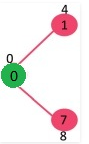Pick the vertex with minimum key value and not already included in MST (not in mstSET). The vertex 1 is picked and added to mstSet. So mstSet now becomes {0, 1}. Update the key values of adjacent vertices of 1. The key value of vertex 2 becomes 8.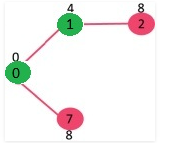Pick the vertex with minimum key value and not already included in MST (not in mstSET). We can either pick vertex 7 or vertex 2, let vertex 7 is picked. So mstSet now becomes {0, 1, 7}. Update the key values of adjacent vertices of 7. The key value of vertex 6 and 8 becomes finite (7 and 1 respectively).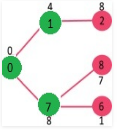Pick the vertex with minimum key value and not already included in MST (not in mstSET). Vertex 6 is picked. So mstSet now becomes {0, 1, 7, 6}. Update the key values of adjacent vertices of 6. The key value of vertex 5 and 8 are updated.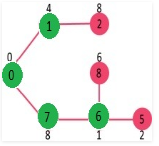We repeat the above steps until mstSet includes all vertices of given graph. Finally, we get the following graph.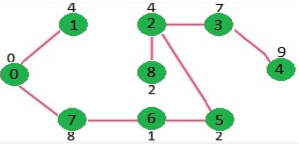How to implement the above algorithm?

We use a boolean array mstSet[] to represent the set of vertices included in MST. If a value mstSet[v] is true, then vertex v is included in MST, otherwise not. Array key[] is used to store key values of all vertices. Another array parent[] to store indexes of parent nodes in MST. The parent array is the output array which is used to show the constructed MST.

// A C / C++ program for Prim's Minimum Spanning Tree (MST) algorithm.
// The program is for adjacency matrix representation of the graph

#include <stdio.h>
#include <limits.h>

// Number of vertices in the graph
#define V 5

// A utility function to find the vertex with minimum key value, from
// the set of vertices not yet included in MST
int minKey(int key[], bool mstSet[])
{
// Initialize min value
int min = INT_MAX, min_index;

for (int v = 0; v < V; v++)
if (mstSet[v] == false && key[v] < min)
min = key[v], min_index = v;

return min_index;
}

// A utility function to print the constructed MST stored in parent[]
int printMST(int parent[], int n, int graph[V][V])
{
printf("Edge   Weight ");
for (int i = 1; i < V; i++)
printf("%d - %d    %d ", parent[i], i, graph[i][parent[i]]);
}

// Function to construct and print MST for a graph represented using adjacency
// matrix representation
void primMST(int graph[V][V])
{
int parent[V]; // Array to store constructed MST
int key[V];   // Key values used to pick minimum weight edge in cut
bool mstSet[V];  // To represent set of vertices not yet included in MST

// Initialize all keys as INFINITE
for (int i = 0; i < V; i++)
key[i] = INT_MAX, mstSet[i] = false;

// Always include first 1st vertex in MST.
key = 0;     // Make key 0 so that this vertex is picked as first vertex
parent = -1; // First node is always root of MST

// The MST will have V vertices
for (int count = 0; count < V-1; count++)
{
// Pick the minimum key vertex from the set of vertices
// not yet included in MST
int u = minKey(key, mstSet);

// Add the picked vertex to the MST Set
mstSet[u] = true;

// Update key value and parent index of the adjacent vertices of
// the picked vertex. Consider only those vertices which are not yet
// included in MST
for (int v = 0; v < V; v++)

// graph[u][v] is non zero only for adjacent vertices of m
// mstSet[v] is false for vertices not yet included in MST
// Update the key only if graph[u][v] is smaller than key[v]
if (graph[u][v] && mstSet[v] == false && graph[u][v] <  key[v])
parent[v]  = u, key[v] = graph[u][v];
}

// print the constructed MST
printMST(parent, V, graph);
}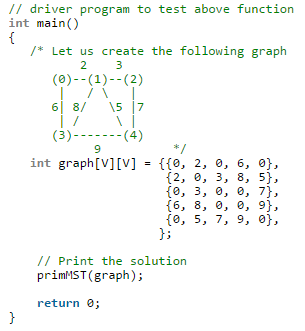Output:
Edge   Weight
0 - 1    2
1 - 2    3
0 - 3    6
1 - 4    5

Time Complexity of the above program is O(V^2). If the input graph is represented using adjacency list, then the time complexity of Prim’s algorithm can be reduced to O(E log V) with the help of binary heap.

Offer running on EduRev: Apply code STAYHOME200 to get INR 200 off on our premium plan EduRev Infinity!

## GATE Computer Science Engineering(CSE) 2022 Mock Test Series

131 docs|167 tests

,

,

,

,

,

,

,

,

,

,

,

,

,

,

,

,

,

,

,

,

,

;